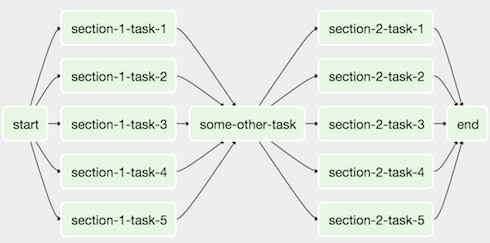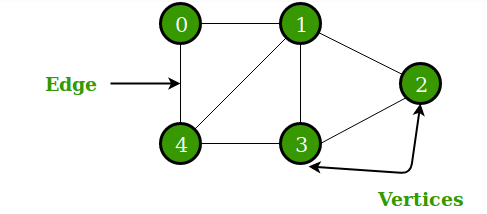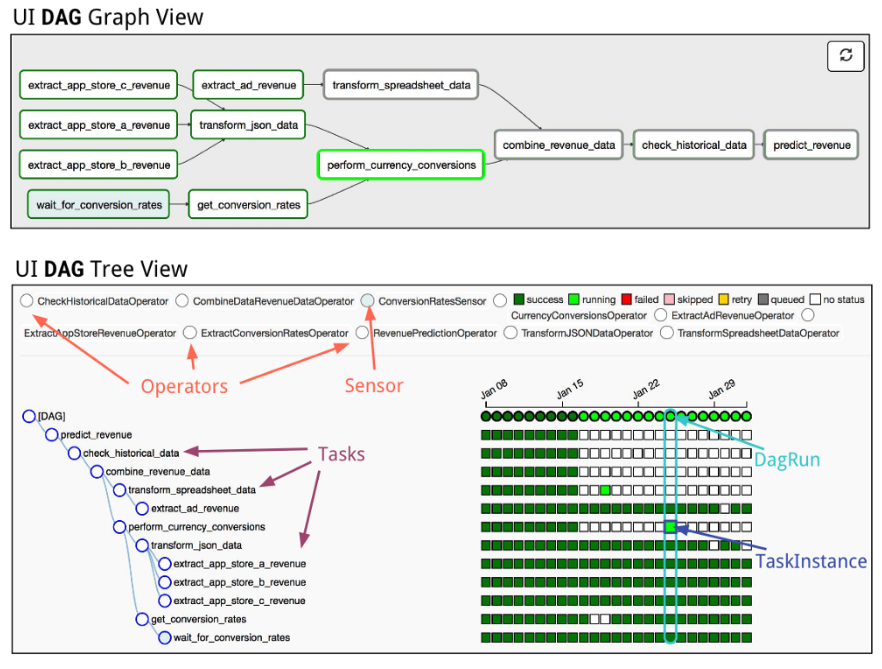# Airflow tutorial 5: Airflow concept

We will learn about Airflow’s key concepts

## Overview

Airflow is a workflow management system which is used to programmatically author, schedule and monitor workflows.

## DAGs

Workflows are called DAGs (Directed Acyclic Graph).

• A DAG is a collection of all the tasks you want to run, organized in a way that reflects their relationships and dependencies.### Understand Directed Acyclic Graph

To understand what is a Directed Acyclic Graph? First, we need to understand the graph data structure. This is a very special data structure in computer science.

A Graph has 2 main parts:

• The vertices (nodes) where the data is stored
• The edges (connections) which connect the nodesGraphs are used to solve many real-life problems because they are used to represent networks.

• For example: social networks, system of roads, airline flights from city to city, how the Internet is connected, etc.
• Undirected graph: The relationship exists in both directions, the edge has no direction. Example: If Mary was a friend of Francis, Francis would likewise be a friend of Mary.

• Directed graph (digraph): Direction matters, since the edges in a graph are all one-way

• An example graph: the course requirements for a computer science major.
• The class prerequisites graph is clearly a digraph since you must take some classes before others.
• Acyclic graph: a graph has no cycles.
• Cyclic graph: a graph has cycles.
• A cycle in a directed graph is a path that starts and ends at the same node.
• For example, the path (V5,V2,V3,V5) is a cycle. This is a loop.

### DAGs Summary

Directed Acyclic Graph is a graph that has no cycles and the data in each node flows forward in only one direction.

It is useful to represent a complex data flows using a graph.

• Each node in the graph is a task
• The edges represent dependencies amongst tasks.
• These graphs are called computation graphs or data flow graphs and it transform the data as it flow through the graph and enable very complex numeric computations.
• Given that data only needs to be computed once on a given task and the computation then carries forward, the graph is directed and acyclic. This is why Airflow jobs are commonly referred to as “DAGs” (Directed Acyclic Graphs)

Beside Airflow, there are other cutting edge big data/data science frameworks is built using graph data structure.

• Tensorflow - An open source machine learning framework
• TensorFlow uses a dataflow graph to represent your computation in terms of the dependencies between individual operations.

• DAGs do not perform any actual computation. Instead, Operators determine what actually gets done.
• Task: Once an operator is instantiated, it is referred to as a “task”. An operator describes a single task in a workflow.
• Instantiating a task requires providing a unique `task_id` and `DAG` container
• A DAG is a container that is used to organize tasks and set their execution context.
``````# t1, t2 are examples of tasks created by instantiating operators
t1 = BashOperator(
bash_command='date',
dag=dag,
)

t2 = BashOperator(
depends_on_past=False,
bash_command='sleep 5',
dag=dag,
)
``````

### Operators categories

Typically, Operators are classified into three categories:

### Working with Operators

• Airflow provides prebuilt operators for many common tasks.
• There are more operators being added by the community. You can just go to the Airflow official Github repo, specifically in the `airflow/contrib/` directory to look for the community added operators.
• All operators are derived from `BaseOperator` and acquire much functionality through inheritance. Contributors can extend `BaseOperator` class to create custom operators as they see fit.
``````class HiveOperator(BaseOperator):
"""
HiveOperator inherits from BaseOperator
"""
``````

• After defining a DAG, and instantiate all the tasks, you can then set the dependencies or the order in which the tasks should be executed.
• Task dependencies are set using:
• the `set_upstream` and `set_downstream` operators.
• the bitshift operators `<<` and `>>`
``````# This means that t2 will depend on t1
# running successfully to run.
t1.set_downstream(t2)

# bit shift operator
# t1 >> t2
``````

• A key concept in Airflow is the `execution_time`. The execution times begin at the DAG’s `start_date` and repeat every `schedule_interval`.
• For this example the scheduled execution times would be (“2018–12–01 00:00:00”, “2018–12–02 00:00:00”, …). For each execution_time, a `DagRun` is created and operates under the context of that execution time. A `DagRun` is simply a DAG that has a specific execution time.
``````default_args = {
'owner': 'airflow',
'start_date': datetime(2018, 12, 01),
# 'end_date': datetime(2018, 12, 30),
'retries': 1,
'retry_delay': timedelta(minutes=5),
}

dag = DAG(
'tutorial',
default_args=default_args,
description='A simple tutorial DAG',
# Continue to run DAG once per day
schedule_interval=timedelta(days=1),
)
``````
• DagRuns are DAGs that runs at a certain time.
• Each DagRun and TaskInstance is associated with an entry in Airflow’s metadata database that logs their state (e.g. “queued”, “running”, “failed”, “skipped”, “up for retry”).Screenshot taken from Quizlet’s Medium post

Tags:

Categories:

Updated: